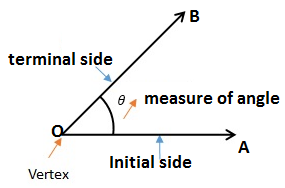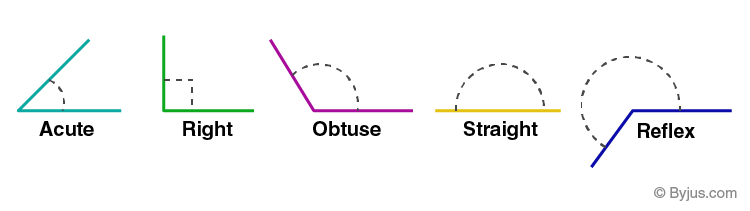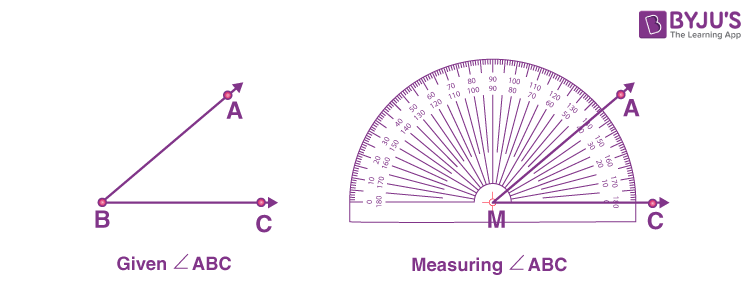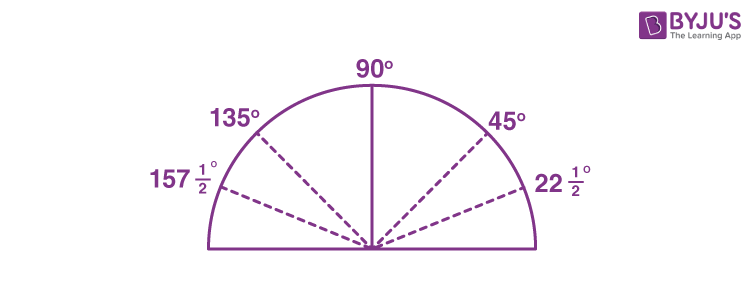# Measuring Angles

Measuring angles is a significant topic in geometry. An angle can be measured using a protractor, precisely. An angle is measured in degrees, hence its called ‘degree measure’. One complete revolution is equal to 360 degrees, hence it is divided into 360 parts. Each part of the revolution is a degree. If we know how to measure angle, then it will be very easy to construct an angle using a protractor.

Any angle whether it is acute, obtuse, reflex, or right angle, can be measured using a protractor. Although based on the right angle, we are able to differentiate between acute, obtuse or reflex angles. But if we have two acute angles to measure, then we cannot determine the greater angle among the two. Therefore, we have to use a protractor to measure the angles accurately.

## What is an Angle?

An angle is a shape in geometry that is formed when two rays are joined together at a single point. The point where two rays meet is called the vertex of the angle and two rays are called the arms of the angle.

Also check: Definition of angleThere are different types of angles based on their measures, such as:

• Acute Angle: Formed between 0° to 90.
• Obtuse Angle: Formed between 90° to 180°
• Right Angle: The angle which is exactly equal to 90°
• Straight Angle: The angle which is exactly equal to 180°
• Reflex Angle: The angle which is greater than 180 degrees and less than 360 degrees
• Full Rotation: The complete rotation of angle equal to 360 degrees## How to Measure angles using Protractor?

A protractor is a semi-circular tool used to draw and measure angles. It is marked with degrees from 0 to 180 degrees. It can be directly used to measure any angle from 0 to 360 degrees. The markings are made in two ways, 0 to 180 degrees from right to left and vice versa.

Suppose we have an angle to measure say, ∠ABC. Follow the steps given below to measure the angle.

Step 1. Put the protractor above the line BC such that the midpoint of protractor is at point B.

Step 2. Adjust the protractor in such a way that BC is parallel to the straight-edge of the protractor.

Step 3. The protractor has two ‘scales’ marked from 0 to 180 degrees on both the ends. Take the reading where BC coincides with the 0 degree.

Step 4. Now from 0 degrees, check the ray BA coincides with the curved edges of the protractor. This reading gives us the measure of angle ABC.### Measuring angle greater than 180 degrees

To measure the angle greater than 180 degrees, we can put the protractor on the arm of the angle upside down and then mark the point on the curved edge of the protractor.

For example, if we have to measure an angle equal to 210 degrees, then put the protractor upside down on the arm of the angle such that the middle point coincides with the vertex of the angle.

Now follow the same steps as we have discussed above for the angles less than or equal to 180 degrees.

 Facts: Angles are measured in degrees or radians. A right angle is ¼ revolution and a straight angle is ½ a revolution A straight angle forms a straight line and both its arms coincides with the straight edges of the protractor When the sum of the measures of two angles is that of a right angle, then each one of them is 45 degrees When the sum of the measures of two angles is that of a straight angle and if one of them is acute then the other should be an obtuse angle.

### How to Measure Angles by Folding Paper?

In Class 6 we were taught how to measure angles using a paper or by paper fold method.

For example, cut a paper in circular shape using a bangle or compass. Fold it once to get the shape of a semicircle. The straight edge of the semicircle formed shows the 180 degree angle. Again fold it into equal half and mark the folded line. Now, mark 90 degrees in this fold.

If we fold the 90 degrees into equal half, then we get an angle equal to 45 degrees. Now if we open the sheet to have the semi circle shape, then the mark opposite to the 45 degrees, across 90 degrees, can be marked as 135 degrees (45 + 90 = 135).

Further, if we fold the 45 degrees mark, then we will get the angle equal to 22 ½ degrees and the angle on the left of 135 degrees will be (135 + 22 ½ = 157 ½ degrees).Now based on these scales starting from 0°, 22½° , 45°, 90°, 135°, 157½° and 180°, we can measure the other angles, approximately.

### Practice Problems

1. Draw the angle of 100 degrees.
2. Draw the angle of 45 degrees using a protractor. Then taking the measure of 45 degrees and without using a protractor draw another angle of 135 degrees. (Hint: use a compass).
3. Give two examples of each:
1. Acute angle
2. Obtuse angle

## Frequently Asked Questions on Measuring Angles

### How should we measure angle accurately?

To measure angles precisely, we need to use a protractor.

### What are the types of angles to be measured?

In geometry, there are basically six types of angles that can be measured using a protractor, they are acute angle, right angle, obtuse angle, straight angle, reflex angle and full rotation.

### What is the symbol for an angle?

An angle is represented by the symbol ∠.

### What is the measure of sum of two right angles?

The measure of angle which is equal to the sum of two right angles is 180 degree angle or straight angle.

### Can we measure the angle greater than 180 degrees using a protractor?

Yes, we can measure the angles greater than 180 degrees using a protractor.